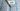# Validate Sudoku - Leet Code Solution

September 03, 2020## Problem Statement

Determine if a 9x9 Sudoku board is valid. Only the filled cells need to be validated according to the following rules:

• Each row must contain the digits 1-9 without repetition.
• Each column must contain the digits 1-9 without repetition.
• Each of the 9 3x3 sub-boxes of the grid must contain the digits 1-9 without repetition.

## Solution

Its a simple solution where we need to verify on two parts:

• Each row and column must have unique value (1-9).
• Each `3x3` board inside the big `9x9` board for the unique value.

### Check for each Row and Column

• We can iterate over matrix and can check a row and column in one iteration pass. Let me try to depict it in the form of some images:• So in first iteration, we will cover single row and single column like this.
• After first iteration, complete matrix will be checked.
• We need to keep track of values for each row and col, for one iteration. So that, we can check if we got a same number or not.
• We can have an array of length-9, since we have numbers from 1-9. Index-0 will be kept unused.

### Code Snippet for this

``````//char[][] board
for (int i=0; i<board.length; i++) {
boolean checkRow[] = new boolean;
boolean checkCol[] = new boolean;

for (int j=0; j<board.length; j++) {
if (board[i][j] != '.') {
//check row
if (checkRow[board[i][j] - '1']) {
return false;
}
else {
checkRow[board[i][j] - '1'] = true;
}
}

if (board[j][i] != '.') {
//check col
if (checkCol[board[j][i] - '1']) {
return false;
}
else {
checkCol[board[j][i] - '1'] = true;
}
}
}
}``````

### Check for each 3x3 matrix

• Similarly, while iterating over matrix. We need to check for each 3x3 matrix.

See the image below for more understanding:Lets look at the code:

``````//char[][] board
for (int i=0; i<board.length; i+=3) {
for (int j=0; j<board.length; j+=3) {
boolean check3x3[] = new boolean;
for (int k=i; k<i+3; k++) {
for (int l=j; l<j+3; l++) {
if (board[k][l] == '.') continue;

if (check3x3[board[k][l] - '1']) {
return false;
}
else {
check3x3[board[k][l] - '1'] = true;
}
}
}
}
}``````

## Final Code

``````public boolean isValidSudoku(char[][] board) {
for (int i=0; i<board.length; i++) {
boolean checkRow[] = new boolean;
boolean checkCol[] = new boolean;

for (int j=0; j<board.length; j++) {
if (board[i][j] != '.') {
//check row
if (checkRow[board[i][j] - '1']) {
return false;
}
else {
checkRow[board[i][j] - '1'] = true;
}
}

if (board[j][i] != '.') {
//check col
if (checkCol[board[j][i] - '1']) {
return false;
}
else {
checkCol[board[j][i] - '1'] = true;
}
}
}
}

for (int i=0; i<board.length; i+=3) {
for (int j=0; j<board.length; j+=3) {
boolean check3x3[] = new boolean;
for (int k=i; k<i+3; k++) {
for (int l=j; l<j+3; l++) {
if (board[k][l] == '.') continue;

if (check3x3[board[k][l] - '1']) {
return false;
}
else {
check3x3[board[k][l] - '1'] = true;
}
}
}
}
}

return true;
}``````

## Complexity

The complexity is `O(mxn)`
We are iterating over whole matrix 2 times. If you are confused for last loop where you can see three nested loops. We are actually iterating over matrix only once.

## Leet code submission result

``````Runtime: 1 ms, faster than 100.00% of Java online submissions for Valid Sudoku.

Memory Usage: 39.5 MB, less than 80.98% of Java online submissions for Valid Sudoku.
``````